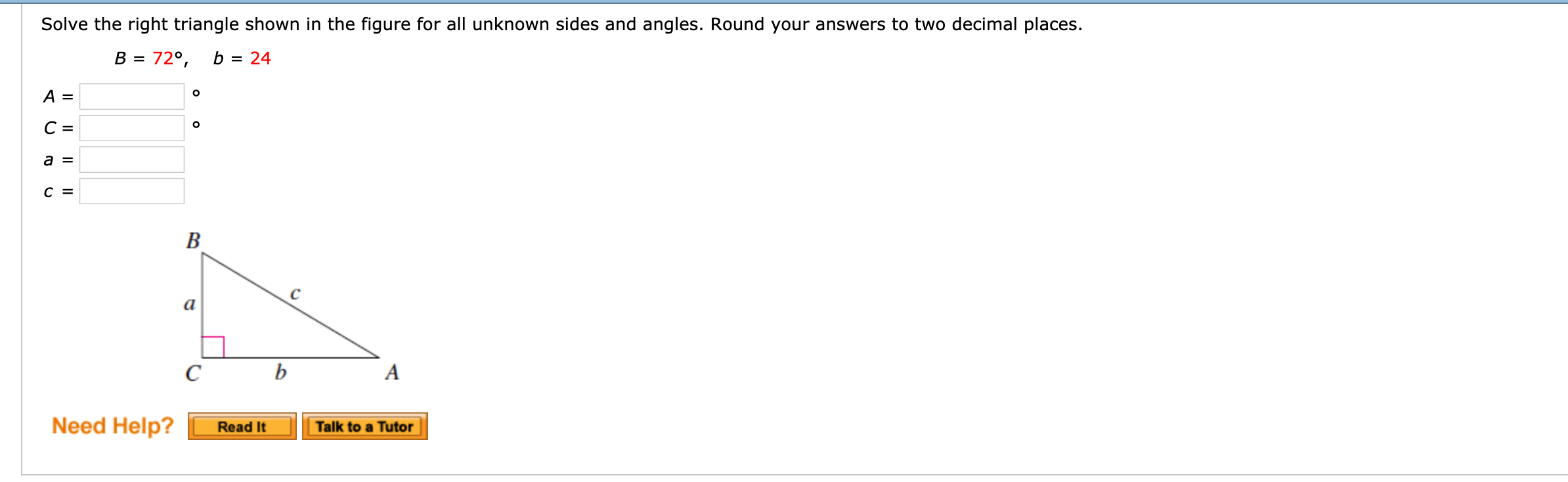# Solve the right triangle shown in the figure for all unknown sides and angles. Round your answers to two decimal places.a FRead It Talk to a TutorNeed Help?

Question
858 viewshelp_outlineImage TranscriptioncloseSolve the right triangle shown in the figure for all unknown sides and angles. Round your answers to two decimal places. a F Read It Talk to a Tutor Need Help? fullscreen
check_circle

star
star
star
star
star
1 Rating
Step 1

Since angle Cis right angle,so, C=90 degrees. we calculate angle A , using the property that sum of angles of triangle is 180 degrees.

Step 2

Then we use sine law to find the remai...

### Want to see the full answer?

See Solution

#### Want to see this answer and more?

Solutions are written by subject experts who are available 24/7. Questions are typically answered within 1 hour.*

See Solution
*Response times may vary by subject and question.
Tagged in
MathCalculus

### Other